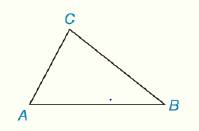Chapter 2.CT, Problem 7CT### Elementary Geometry for College St...

6th Edition
Daniel C. Alexander + 1 other
ISBN: 9781285195698

#### Solutions

Chapter
Section### Elementary Geometry for College St...

6th Edition
Daniel C. Alexander + 1 other
ISBN: 9781285195698
Textbook Problem
1 views

# For Δ A B C , find m ∠ B ifa) m ∠ A = 65 ° and m ∠ C = 79 ° _______________.b) m ∠ A = 2 x , m ∠ B = x , and m ∠ C = ( 2 x + 15 ) ________.To determine

a)

To find:

The value of mB.

Explanation

Given:

The following figure shows the given diagram.

mA=65° and mC=79°.

Note:

The sum of interior angle of any triangle will be 180°.

Calculation:

Equation for sum of interior angle of a triangle is as follow,

mA+mB+mC=180°

Substitute 65° for the mA, and 79° for mC in the above equation to obtained the value of mB

To determine

b)

To find:

The value of mB.

### Still sussing out bartleby?

Check out a sample textbook solution.

See a sample solution

#### The Solution to Your Study Problems

Bartleby provides explanations to thousands of textbook problems written by our experts, many with advanced degrees!

Get Started

#### Find more solutions based on key concepts